Get Started »

# Geometry

The Graphics command generates all kinds of 2D shapes:

 In:=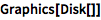⨯ `Graphics[Disk[]]`
 Out=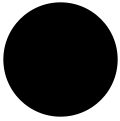Many geometric objects take a series of coordinates (lists) as arguments:

 In:=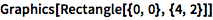⨯ `Graphics[Rectangle[{0, 0}, {4, 2}]]`
 Out=Pass in a list with directives to combine graphics and change their styles:

 In:=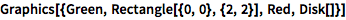⨯ `Graphics[{Green, Rectangle[{0, 0}, {2, 2}], Red, Disk[]}]`
 Out=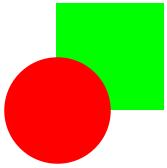Generate triangles with commands like SASTriangle:

(Type ESCdegESC for the `°` symbol.)
 In:=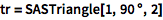⨯ `tr = SASTriangle[1, 90 \[Degree], 2]`
 Out=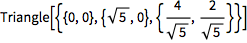Properties like area can be computed directly:

 In:=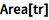⨯ `Area[tr]`
 Out=Pass this expression into Graphics:

 In:=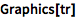⨯ `Graphics[tr]`
 Out=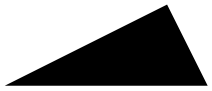Similarly, you can use Graphics3D to display 3D objects:

 In:=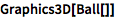⨯ `Graphics3D[Ball[]]`
 Out=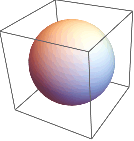Calculate volume and other properties:

(With no arguments given, a cylinder has a radius of 1 and a height of 2.)
 In:=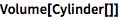⨯ `Volume[Cylinder[]]`
 Out=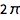You can also find formulas and other information using natural-language input:

 In:=X `volume of a cone`
 Out=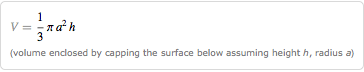Geometric transformations like Rotate, Translate and Scale are built in:

 In:=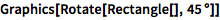⨯ `Graphics[Rotate[Rectangle[], 45 \[Degree]]]`
 Out=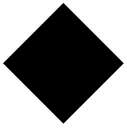QUICK REFERENCE: Plane Geometry `»`

QUICK REFERENCE: Solid Geometry `»`

QUICK REFERENCE: Geometric Transforms `»`